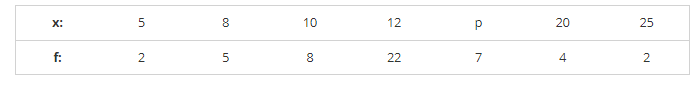# Find the missing value of p for the following distribution whose mean is 12.58.

Question:

Find the missing value of p for the following distribution whose mean is 12.58.Solution:It is given that,

Mean = 12.58

$\Rightarrow \sum f_{X} / N=12.58$

$\Rightarrow \frac{7 p+524}{50}=12.58$

⇒ 7p + 524 = 629

⇒ 7p = 629 − 524

⇒ 7p = 105

⇒ p = 1057 = 15

⇒ p = 15

∴ p = 18.